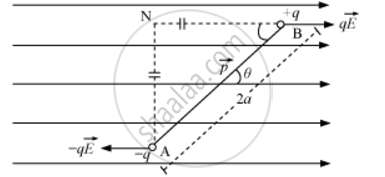Share

# Deduce the Expression for the Torque Acting on a Dipole of Dipole Moment in the Presence of a Uniform Electric Field - Physics

#### Question

Deduce the expression for the torque acting on a dipole of dipole moment vecp in the presence of a uniform electric field vecE

#### Solution

Dipole in a Uniform External FieldConsider an electric dipole consisting of charges −q and +q and of length 2a placed in a uniform electric field vecEmaking an angle θ with electric field.

Force on charge -q" at "A=-qvecE("opposite to" vecE)

Force on charge +q" at "B=qvecE("along"vecE)

Electric dipole is under the action of two equal and unlike parallel forces, which give rise to a torque on the dipole.

τ = Force × Perpendicular distance between the two forces

τ = qE (AN) = qE (2a sin θ)

τ = q(2a) E sinθ

τ = pE sinθ

:.vect=vecpxxvecE

Is there an error in this question or solution?